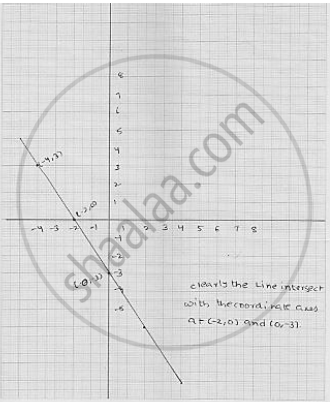# Draw the Graph of the Equation Given Below. Also, Find the Coordinate of the Points Where the Graph Cuts the Coordinate Axes : 3x + 2y + 6 = 0 - Mathematics

Draw the graph of the equation given below. Also, find the coordinate of the points
where the graph cuts the coordinate axes : 3x + 2y + 6 = 0

#### Solution

We have

3x + 2y + 6 = 0

⇒   2y = - 6 - 3x

⇒    y = (-6 -3x) / 2

Putting  x = - 2 in (1) , we get  x =( 6-3 (-2)) /2= 0

Putting  x = - 4 in (1) , we get  y = (6 - 3 (-4)) /2 = 3

Thus, we obtain the following table giving coordinates of two points on the line
represented by the equation   3x + 2y + 6 = 0

Graph of the equation   3x + 2y + 6 = 0Concept: Graph of a Linear Equation in Two Variables
Is there an error in this question or solution?

#### APPEARS IN

RD Sharma Mathematics for Class 9
Chapter 7 Linear Equations in Two Variables
Exercise 7.3 | Q 12.4

Share